# Complex Analysis : Complex Integration

## Example Questions

### Example Question #1 : Complex Integration

Letbe the region of the complex plane enclosed by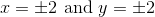Compute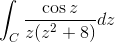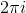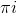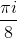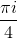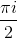Explanation:

Recall Cauchy's integral formula, which states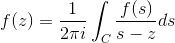In this case, we have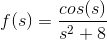Plugging in gives us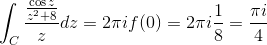### Example Question #1 : Complex Integration

Letbe the unit circle.

Compute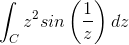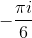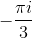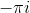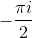Explanation:

Recall the Taylor expansion of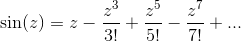Use this to write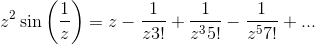The coefficient of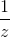is the residue, so by the residue theorem, the value of the integral is### All Complex Analysis Resources